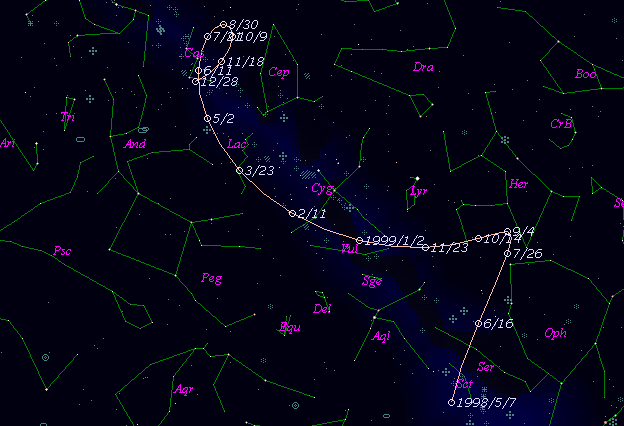# \$B%j%K%"WB@1(B

C/1998 M2 ( LINEAR )###\$B%W%m%U%#!<%k(B

 \$BH/8+F|(B 1998\$BG/(B6\$B7n(B19\$BF|(B \$BH/8+8wEY(B 16.9\$BEy(B \$BH/8+ Lincoln Laboratory Near-Earth Asteroid Research project

###\$B###\$B50F;MWAG(B

```   The following improved orbital elements by Kenji Muraoka, are
from 357 observations 1998 May 28 to 1999 Aug. 12, perturbations
by 9 Planets, Moon and 5 minor planets were taken into account.
The mean residual is +/- 0.62 arc seconds.

Epoch  =  1998 Aug. 15.0  TT       JDT = 2451040.5
T  =  1998 Aug. 13.17111       +/- 0.00055 (m.e.) TT
Peri. =   37.24261                +/- 0.00017
Node  =  260.88023                +/- 0.00011   (2000.0)
Incl. =   60.18235                +/- 0.00005
q  =    2.7253289              +/- 0.0000036 AU
e  =    0.9977532              +/- 0.0000053
1/a  =   +0.0008244              +/- 0.0000020 1/AU
( P  =  42246 years )
orig. 1/a  =   +0.001223
fut.  1/a  =   +0.001871
```

###\$B@1?^(B###\$B8wEYJQ2=(B

```        m1 = 8.0 + 5 log\$B&\$(B + 10.0 log r
```##### \$B50F;MWAG\$OB<2,7r<#;a\$N7W;;\$K\$h\$k\$b\$N\$G\$9!#(B \$B@1?^\$O(B StellaNavigator Ver.2.0 for Windows (\$B%"%9%H%m%"!<%D(B \$BJTCx(B / \$B%"%9%-!<=PHG6I4)(B) \$B\$G:n@.\$7\$?\$b\$N\$G\$9!#(B \$B8wEY%0%i%U\$O(BComet for Windows\$B\$G:n@.\$7\$?\$b\$N\$G\$9!#(B